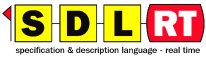## 12 - Symbols contained in diagrams

The table below shows what symbols can be contained in a specific diagram type.
 In the diagrams listed in this column the ticked symbols on the right can be used. package block class process class block process procedure declaration semaphore declaration channel additional heading text gate definition gate usage behavior symbols package x x x x x x x x x x x - - block class - x x x x x x x x x x x - process class - - - - - - - - x x x - x block - - - x x x x x x x - x - process - - - - - - - - x x - - x procedure - - - - - - - - - x - - x

A diagram listed in the first column can contain the ticked symbols in the other columns. For example the process symbol can contain the additional heading symbol, the text symbol and all the behavior symbols. The behavior symbols are all symbols described in "Behavior" on page 13.